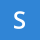Home
IT Knowledge
Inspiration
Languages
EN

# Java 8 - difference between lambda expression and method reference

0 points
Created by:Sylvie-Justice
280

In this article, we would like to show you the differences between lambda expression and method reference in Java 8.

## 1. Overview

In this section, we list a few general differences.

Lambda expression:

• an anonymous method (it doesn't have a name),
• is used to provide the inline implementation of a method defined by the functional interface,
• the arrow operator (`->`) connects the argument and functionality,
• long lambda expressions consisting of many statements may reduce the readability of the code.

Method reference:

• re-usable (no need to copy and paste lambda expression),
• refers to a method without executing it,
• the double colon operator  (`::`) separates the method name from the name of an object or class,
• extracting many statements from lambda expression in a method and referencing it may improve the readability of the code,

## 2. Syntax

Lambda ExpressionMethod Reference

One parameter:

``parameter -> expression``

Multiple parameters:

``(parameter1, parameter2, parameterN) -> expression``

For more complex operations:

``(parameter1, parameter2, parameterN) -> { code block }``

Reference to a static method:

``ContainingClass::staticMethodName  ``

Reference to an instance method of a particular object:

``containingObject::instanceMethodName``

Reference to an instance method of an arbitrary object of a particular type:

``ContainingType::methodName``

Reference to a constructor:

``ClassName::new``

### Practical example

In this example, we use both lambda expression and method reference to achieve the same effect - print `list` items in lowercase.

``````import java.io.*;
import java.util.ArrayList;
import java.util.List;

public class Example {

public static void main(String[] args) throws IOException {
List<String> list = new ArrayList<>();

// Using lambda expression to print list items in lower case
System.out.println("----- Lambda expression -----");
list.stream().map(x -> x.toLowerCase())
.forEach(x -> System.out.println(x));

// Using method reference to print list items in lower case
System.out.println("----- Method reference -----");
list.stream().map(String::toLowerCase).sorted()
.forEach(System.out::println);
}
}``````

Output:

``````----- Lambda expression -----
a
b
c
----- Method reference -----
a
b
c``````